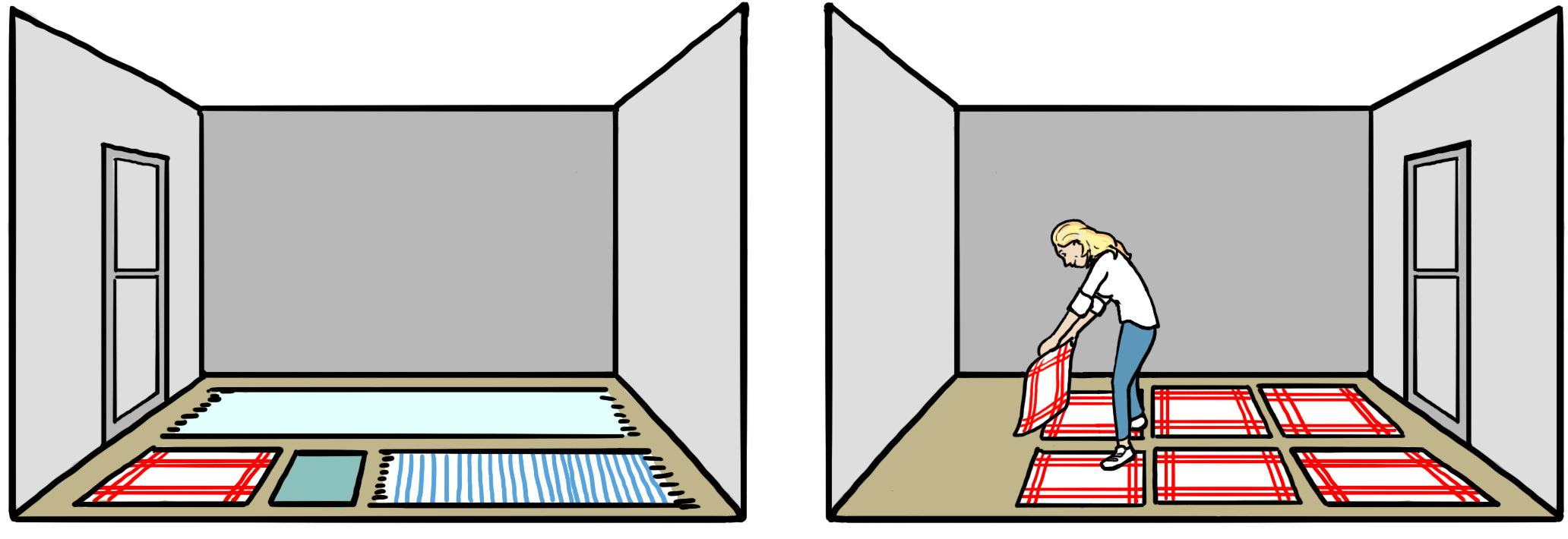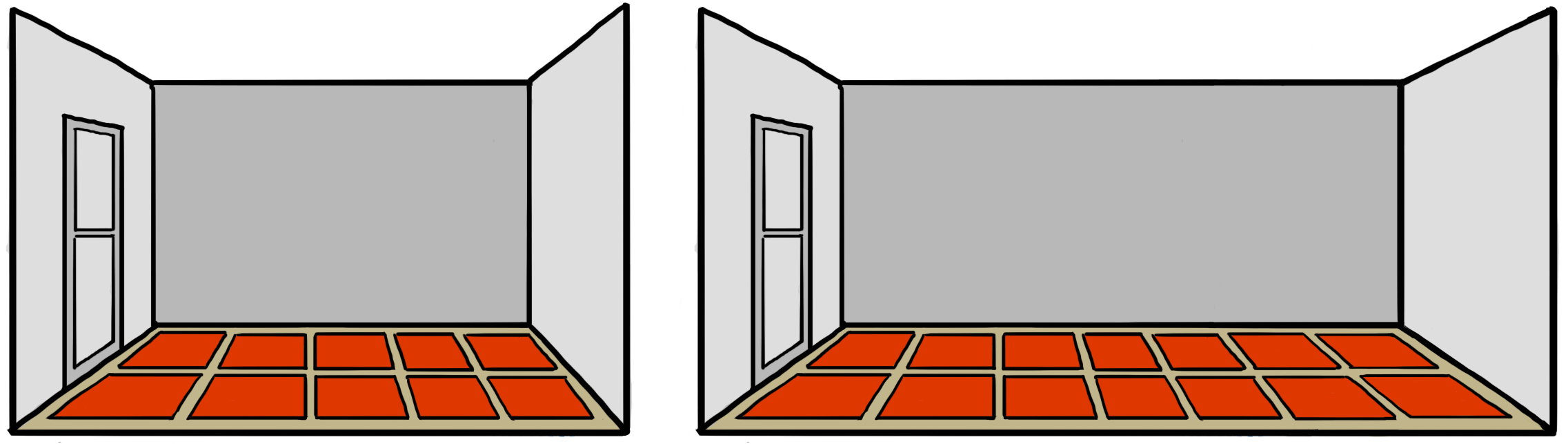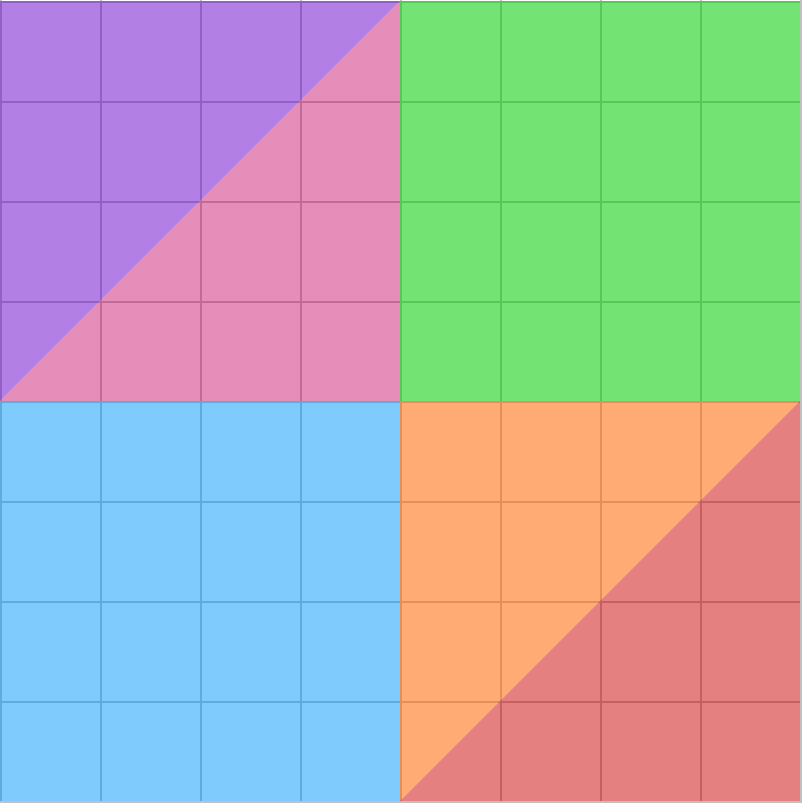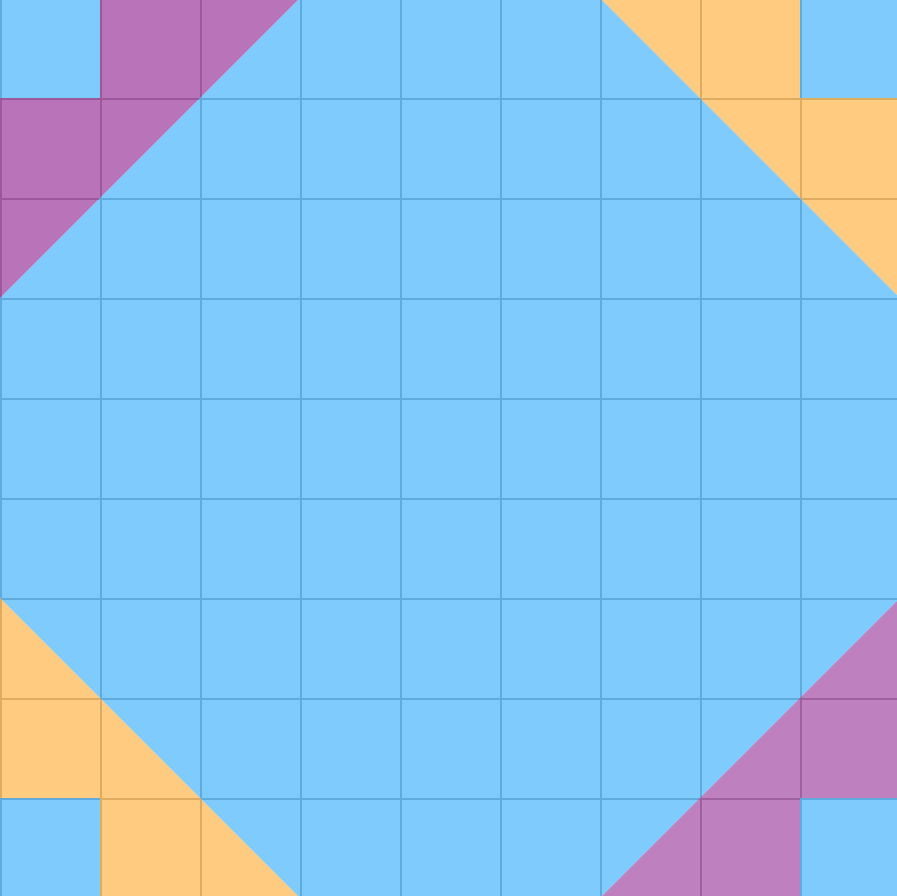# What Are the Metric Units for Area?

This entry uses the metric system. For the imperial system click here.

When you want to describe the size of a surface, you are talking about an area. Examples of this are the size of a floor, a piece of paper, or a wall. How many doormats can you fit on the floor of your living room? How many pieces of paper can you fit in the hallway? How many towels can you fit on the floor of your bedroom?Because towels can come in many different sizes, it can be difficult to agree on how big a surface is if you measure it in the number of towels you can put on it. Which towel should you use? A bath towel or a kitchen towel? Because there isn’t any standard towel size, we agreed to use a square with one meter on each side. We call this square a square meter. Because we all agree on the length of a meter, we also agree on the size of the square meter.Area is measured in square meters (m2). A square meter is as large as a square where every side has a length of one meter. If you want to measure the area of smaller things, you can use square centimeters (cm2). Below you can see an example of an area with a size of $1$ m2.You can also have surfaces with an area of $1$ cm2. The figure below is an example of this. It has a length and width of $1$ cm2 each.You might have already learned that $1$ m is the same as $100$ cm. But $1$ m2 is not the same as $100$ cm2. There’s actually space for $10\phantom{\rule{0.17em}{0ex}}000$ squares of $1$ cm2 in the first figure with size $1$ m2. Take a look at the figure below. You can see that the blue square ($1$ cm2) can fit $100$ times into the white square. The white square can fit $100$ times into the pink square, which has the size $1$ m2. That means that there is space for $100\cdot 100=10\phantom{\rule{0.17em}{0ex}}000$ of the blue squares ($1$ cm2) in the pink square of $1$ m2.Example 1

Eric is going to paint the pattern below on one of the walls at his school, and he has to calculate how much paint he needs.If every square is $\text{}1\text{}\phantom{\rule{0.17em}{0ex}}{\text{m}}^{2}$, how many square meters of paint does he need for every color?

Eric needs $8$ m2 of purple paint, $8$ m2 of pink paint, $8$ m2 of orange paint, $8$ m2 of red paint, $16$ m2 of blue paint and $16$ m2 of green paint.

Example 2

Cathy needs to cut out the pattern below from colored pieces of paper.How many square centimeters of paper does she need of each color if each square is $\text{}1\text{}\phantom{\rule{0.17em}{0ex}}{\text{cm}}^{2}$?

Cathy needs $7$ cm2 of yellow paper, $7$ cm2 of purple paper and $67$ cm2 of blue paper.

So, if you want to measure smaller surfaces, you can use square decimeters, square centimeters or square millimeters. If you want to measure larger surfaces, you can use square kilometers. As one meter is ten decimeters, one square decimeter (dm2) is a square with sides equal to one tenth of a meter (a decimeter). You might think that one square meter has space for ten square decimeters. But that’s not the case! You can fit $100$ square decimeters in one square meter. You can see the reason for this in the figure below.

In the same way, one square centimeter (cm2) is a square where each side has a length of one hundredth of a meter (one centimeter). As there are $100$ cm in a meter, you can fit a whole $100\cdot 100=10\phantom{\rule{0.17em}{0ex}}000$ square centimeters in a square meter.Similarly, one square millimeter (mm2) is a square where each side has a length of one thousandth of a meter (one millimeter). As there are $1000$ mm in a meter, you can fit a whole $1000\cdot 1000=1\phantom{\rule{0.17em}{0ex}}000\phantom{\rule{0.17em}{0ex}}000$ square millimeters in a square meter!When you need to measure the surface of really big surfaces, for example cities and countries, it can be smart to use larger units. In that case, the unit square kilometers (km2) is often used. A square kilometer is a square where each side has a length of a thousand meters (one kilometer).Notice how I write and do calculations with units. I begin by writing down the expression. Then I check the bubble figure to see what I have to divide or multiply by to get to the unit the exercise asks for. Then, I solve the exercise step by step. The trick is to switch the unit in the expression with this: The multiplication or division sign (depending on which way I’m moving in the bubble figure), the number the bubble figure shows me, and the corresponding unit. In that way, I can work my way through the bubble figure until I get where I need to go.

Example 3

Square meters to square decimeters:

 $4\phantom{\rule{0.17em}{0ex}}{\text{m}}^{2}=\left(4\cdot 100\right)\phantom{\rule{0.33em}{0ex}}{\text{dm}}^{2}=400\phantom{\rule{0.17em}{0ex}}{\text{dm}}^{2}$

Square millimeters to square centimeters:

 $4000\phantom{\rule{0.17em}{0ex}}{\text{mm}}^{2}=\left(4000÷100\right)\phantom{\rule{0.33em}{0ex}}{\text{cm}}^{2}=40\phantom{\rule{0.17em}{0ex}}{\text{cm}}^{2}$

Square decimeters to square centimeters:

 $40\phantom{\rule{0.17em}{0ex}}{\text{dm}}^{2}=\left(40\cdot 100\right)\phantom{\rule{0.33em}{0ex}}{\text{cm}}^{2}=4000\phantom{\rule{0.17em}{0ex}}{\text{cm}}^{2}$

Square centimeters to square decimeters:

 $400\phantom{\rule{0.17em}{0ex}}{\text{cm}}^{2}=\left(400÷100\right)\phantom{\rule{0.33em}{0ex}}{\text{dm}}^{2}=4\phantom{\rule{0.17em}{0ex}}{\text{dm}}^{2}$

Square centimeters to square millimeters:

 $400\phantom{\rule{0.17em}{0ex}}{\text{cm}}^{2}=\left(400\cdot 100\right)\phantom{\rule{0.33em}{0ex}}{\text{mm}}^{2}=40\phantom{\rule{0.17em}{0ex}}000\phantom{\rule{0.17em}{0ex}}{\text{mm}}^{2}$

Square decimeters to square meters:

 $400\phantom{\rule{0.17em}{0ex}}{\text{dm}}^{2}=\left(400÷100\right)\phantom{\rule{0.33em}{0ex}}{\text{m}}^{2}=4\phantom{\rule{0.17em}{0ex}}{\text{m}}^{2}$

Math Vault

Would you like to solve exercises about area? Try Math Vault!